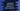# JavaScript program to find the sum of all even numbers below a given number## JavaScript program to find the sum of all even numbers below a given number:

In this JavaScript program, we will learn how to find the sum of all even numbers from 1 to that number. We will use a loop to iterate through the numbers from 1 to that number and for each number, we will check if it is even by using a separate function. If it is even, we will add it to a separate variable that will hold the sum. Finally, it will print the sum.

### Method 1: By using a for loop:

Below is the complete JavaScript program:

``````function isEven(n) {
return n % 2 == 0;
}

function findSum(no) {
let sum = 0;

for (var i = 1; i <= no; i++) {
if (isEven(i)) {
sum += i;
}
}
return sum;
}

console.log(findSum(100));``````

Here,

• isEven method is used to check if a number is even or not. It checks if the number is perfectly divisible by 2.
• The findSum method takes the number as parameter and finds the sum of all even numbers from 1 to that number.
• It uses one for loop that runs from 1 to no and for each number it checks if it is even or not. If it is even, it is adding that value to sum. sum is initialized as 0. So, once the for loop ends, it will hold the total sum of all even numbers in this range.

If I execute the above program, it will give the below output:

``2550``

### Method 2: By using a while loop:

We can also use a while loop to solve it. The below program does that:

``````function isEven(n) {
return n % 2 == 0;
}

function findSum(no) {
let sum = 0;
let i = 1;

while (i <= no) {
if (isEven(i)) {
sum += i;
}
i++;
}
return sum;
}

console.log(findSum(100));``````
• It is similar to the above program.
• The difference is that we are using a while loop. It runs from i=1 to i=no.
• It is working similar to the for loop.